3.03万

# 第24集 大雨中的对峙

00:00
08:42

1、培养孩子们的领导能力；

2、培养孩子们信任与合作的意识；

3、突破思维定式，培养创新与风险意识。6642订阅    20

## 60条评论

•#### 1352569tnvw

不收费就好听
回复
2020-01-16 09:59

春来发几枝_nj 回复 @1352569tnvw: 线圈本少爷啊好吧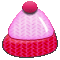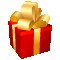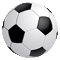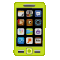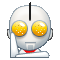•#### 1526983owpg

僵尸先生僵尸先生👮👮👮👮👮👮👮👮👮👮👮👮👮👮👮👮👮👮👮👮👰👰👰👰👰🕴🕴🕴🕴🕴🕴🕴😂😂😂😊😍🤔😌😌😌😉😌😌🤔😝😔😌😌🤔😃😌😌😔😁😌🤔😌😌😍😍🤔你说说你兴农哦住互互双击谢谢明年KTV的时候再见了我们的爱你的手机壳壳牌子好老火车站里面的人的事都在干嘛呐呐喊声明早牛回信息嘻嘻嘻名工哦你一应用😘😍😌😍😍😍😍😍😍😍😍😌😌🙄🙄😁😘😒🤕😘😝😒😃😒🙂😌😌😌🤔😔😌😌😭😍🙂😝🤔😍😌😌🤔😒😌😌😝😌😌😌
回复
2020-03-16 21:04

听友55520498 回复 @1526983owpg: 战斗、男孩、朋友、森林、特种兵、军队

•#### 多晴空

66666666666666666666666666666666666666666666666666666666666666666666666666666666666666666666666666666666666666666666666666666666666666666666666666666666666666666666666666666666666666666666666666666666666666666666666666666666666666666666666666666666666666666666666666666666666666666666666666666666666666666666666666666666666666666666666666666666666666666666666666666666666666666666666666666666666666666666666666666666666666666666666666666666666666666666666666666666666666666666666666666666666666666666666666666666666666666666666666666666666666666666666666666666666666666666666666666666666666666666666
回复
2020-04-14 12:50
•#### 都长命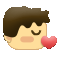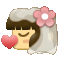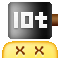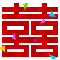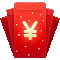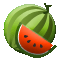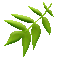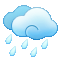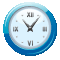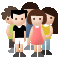回复
2020-03-07 20:27
•#### 听友197804709

^_^^_^^_^^_^^_^^_^^_^^_^^_^^_^^_^^_^^_^^_^^_^^_^^_^^_^^_^^_^^_^^_^^_^^_^^_^^_^^_^^_^^_^^_^^_^^_^^_^^_^^_^^_^^_^^_^^_^^_^^_^^_^^_^^_^^_^^_^^_^^_^^_^^_^^_^^_^^_^^_^^_^^_^^_^^_^^_^^_^^_^^_^^_^^_^^_^^_^^_^^_^^_^^_^^_^^_^^_^^_^^_^^_^^_^^_^^_^^_^^_^^_^^_^^_^^_^^_^^_^^_^^_^^_^^_^^_^^_^^_^^_^^_^^_^^_^^_^^_^
回复
2020-02-16 19:23